### Home > CALC > Chapter Ch2 > Lesson 2.1.1 > Problem2-11

2-11.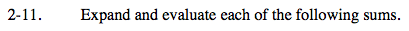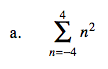(-4)² + (-3)² + (-2)² + (-1)² + (0)² + (1)² + (2)² + (3)² + (4)² = ___

or, because of the symmetry of n2, this problem can be simplified as:

$=2\sum_{-4}^{0}n^{2}=2\left ( (-4)^{2}+(-3)^{2} +(-2)^{2}+(-1)^{2}\right )=2(30)=60$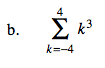Think about the symmetry of odd functions such as k³.
Justify why the sum from −4 to +4 will be 0.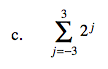Unfortunately, 2j does not have symmetry across the y-axis. We have to expand and evaluate all terms.
= 2 −3 + 2 −2 + ... + 22 + 21 = _______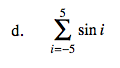sin x is an odd function. Consider symmetry before evaluating.